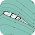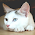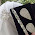# Lid-driven cavity unsteady solution - stream function-vorticity formulation

The lid-driven cavity problem is introduced in the section "Lid-driven cavity flow". In this sub-section, the problem solution using stream function-vorticity formulation is explained.

The stream function can be introduced as follows:
\begin{equation} u = \frac{\partial \psi}{\partial y} \ \ , \ \ v = - \frac{\partial \psi}{\partial x} \end{equation} Using the definition of the stream function and applying it on the continuity equation, we will have:
\begin{equation} \frac{\partial}{\partial x}\frac{\partial \psi}{\partial y} - \frac{\partial}{\partial y} \frac{\partial \psi}{\partial x} = 0 \end{equation} which means that the continuity equation is already verified. Moreover, the vorticity in terms of $u$ and $v$ can be written as:
\begin{equation} \omega = \frac{\partial v}{\partial x} - \frac{\partial u}{\partial y} \end{equation} Now applying:
\begin{equation} -\frac{\partial}{\partial y}\left(\text{x-Momentum}\right) + \frac{\partial}{\partial x}\left(\text{y-Momentum}\right) \end{equation} the momentum equation will be converted to:
\begin{equation} \frac{\partial \omega}{\partial t} + u \frac{\partial \omega}{\partial x} + v \frac{\partial \omega}{\partial y} = \frac{1}{\text{Re}} \nabla^2 \omega \end{equation} On the other hand, vorticity can be written in terms of stream function:
\begin{equation} \omega = \frac{\partial v}{\partial x} - \frac{\partial u}{\partial y} = - \frac{\partial^2 \psi}{\partial x^2} - \frac{\partial^2 \psi}{\partial y^2} \end{equation} So we will have:
\begin{equation} \nabla^2 \psi = -\omega \end{equation} Therefore, the governing equations can be written as follows:
\begin{equation} \left\lbrace{\begin{array}{l} \nabla^2 \psi = -\omega \\ \ \\ \frac{\partial \omega}{\partial t} + u \frac{\partial \omega}{\partial x} + v \frac{\partial \omega}{\partial y} = \frac{1}{\text{Re}} \nabla^2 \omega \end{array}}\right. \end{equation}

# Boundary conditions

The boundary conditions have to be rewritten in terms of vorticity and stream function:
\begin{equation} \left\lbrace{\begin{array}{l} \text{right}\\ \text{left} \end{array}}\right. : u = 0 \Longrightarrow \frac{\partial \psi}{\partial y} = 0 \Longrightarrow \psi = \text{const.} \end{equation}
\begin{equation} \left\lbrace{\begin{array}{l} \text{Top}\\ \text{Bottom} \end{array}}\right. : v = 0 \Longrightarrow \frac{\partial \psi}{\partial x} = 0 \Longrightarrow \psi = \text{const.} \end{equation} It means that everywhere at the boundaries $\psi$ is constant. Since the definition of $\psi$ is based on the derivatives, we are free to choose the constant value, and for simplicity we can set it to $\psi = 0$.

But still there are some boundary conditions to satisfy:
\begin{equation} \left\lbrace{\begin{array}{l} \text{right}\\ \text{left} \end{array}}\right. : v = 0 \Longrightarrow \frac{\partial \psi}{\partial x} = 0 \ ; \ \frac{\partial^2 \psi}{\partial x^2} + \frac{\partial^2 \psi}{\partial y^2} = \frac{\partial^2 \psi}{\partial x^2} = - \omega \end{equation}
\begin{equation} \left\lbrace{\begin{array}{l} \text{bottom} : u = 0 \Longrightarrow \frac{\partial \Psi}{\partial y} = 0 \\ \text{left} \ : u = U_{lid} \Longrightarrow \frac{\partial \Psi}{\partial y} = U_{lid} \end{array}}\right. \Longrightarrow \frac{\partial^2 \Psi}{\partial x^2} + \frac{\partial^2 \Psi}{\partial y^2} = \frac{\partial^2 \Psi}{\partial y^2} = - \omega \end{equation} and the same $\omega$ values for the other boundaries. Fig. d.1.1 shows the boundary conditions for each edge of the cavity.

Fig. d.1.1. Boundary conditions.

# Numerical scheme

To solve the defined problem, a finite difference centred scheme is employed for 1st- and 2nd-order derivatives in space. The uniform grid in time and space is used. A 1st-order scheme in time is implemented (explicit Euler), and a collocation approach is employed so that no staggering is needed. The following steps describe the solution method:

1st step

Solution of the stream function with a SOR method (successive over relaxation):
\begin{equation} \psi_{i,j}^{k+1} = \frac{\alpha}{4}\left(\psi_{i+1,j}^k + \psi_{i-1,j}^k + \psi_{i,j+1}^k + \Delta x \Delta y \omega_{i,j}^n\right) + (1-\alpha)\psi_{i,j}^k \end{equation} where $\alpha$ is the relaxation parameter to be chosen according to the problem. It is clear that at the boundaries $\psi = 0$.

2nd step

Calculating $\omega$ at the boundaries:
\begin{equation} \left\lbrace{\begin{array}{l} \omega_{\text{top}} = \left(\psi_{i,N_y} - \psi_{i,N_y-1}\right) \frac{2}{\Delta y} - U_{lid} \frac{2}{\Delta y} + \mathcal{O}(\Delta y) \\ \ \\ \omega_{\text{bot}} = \left(\psi_{i,1} - \psi_{i,2}\right) \frac{2}{\Delta y} + \mathcal{O}(\Delta y) \\ \ \\ \omega_{\text{lef}} = \left(\psi_{1,j} - \psi_{2,j}\right) \frac{2}{\Delta x} + \mathcal{O}(\Delta x) \\ \ \\ \omega_{\text{rig}} = \left(\psi_{N_x,j} - \psi_{N_x-1,j}\right) \frac{2}{\Delta x} + \mathcal{O}(\Delta x) \end{array}}\right. \end{equation}
3rd step

Solving the vorticity for internal nodes:
\begin{equation} \begin{split} \omega_{i,j}^{n+1} = \omega_{i,j}^n - \Delta t \left[ \right.& \left(\frac{\psi_{i,j+1}^n - \psi_{i,j-1}^n}{2 \Delta y}\right) \left(\frac{\omega_{i+1,j}^n - \omega_{i-1,j}^n}{2 \Delta x}\right) - \\ & \left(\frac{\psi_{i+1,j}^n - \psi_{i-1,j}^n}{2 \Delta x}\right) \left(\frac{\omega_{i,j+1}^n - \omega_{i,j-1}^n}{2 \Delta y}\right) + \\ & \left. \frac{1}{\text{Re}} \left(\frac{\omega_{i+1,j}^n + \omega_{i-1,j}^n + \omega_{i,j+1}^n + \omega_{i,j-1}^n - 4\omega_{i,j}^n}{\Delta x \Delta y}\right) \right] \end{split} \end{equation}

# Numerical results

Fig. d.1.2 shows the results (stream function) for the $\text{Re} = 500$, with the grid spacing of $41 \times 41$. It should be mentioned that for other grid spacing and Reynolds numbers, it is necessary to modify the relaxation factor $\alpha$.

Fig. d.1.2. Numerical results (stream function) for the lid-driven cavity using stream function-vorticity formulation.

The relevant Matlab codes can be downloaded using the following link:
Matlab Codes Bank

#### 7 comments:

1.How can I satisfy the boundary condition at TOP in the code? dphi/dy = Ulid

1.Hi Victor,

the boundary conditions are applied on the streamfunction considering that the cavity contour is a streamline for the flow, therefore its streamfunction value is constant along the walls (and being it arbitrary we set it to zero).

For being consistent, the BC at the top wall for vorticity is

omega(2:Nx-1,Ny) =
- 2*psi(2:Nx-1,Ny-1)/(delta_y^2) - U_top*2/delta_y;

where it is already included that dpsi/dy = U_top.

As you notice, the algorithm does never change the streamfunction value at the boundary, keeping it constantly equal to zero throughout the whole simulation.

Francesco

2.Hi there,

Thanks for the outlined information. Could you tell me for unsteady calculations, what will be an appropriate way for defining the convergence criterion, please?

Best wishes,

3.Hi, I'm working in the lid driven cavity flow using this formulation (stream-function, vorticity), but at the moment I haven't found any info on how to do a stability analysis on the numerical solution. Does you guys have any idea where can I found some info about it?

4.Hi, i'm working in lid driven cavity using this formulation but i can't gets the temperature graphs .
all walls are stationary but top wall is moveing .after dimensionless the top wall temperature is 1.
other wall temperature is zero.
kindly tell the boundary condition is how to write

5.Hi every one, i'm working on the cavity problem with the finite volume method, but the results of the code, aren't consistent with the results by other method like finite difference (vorticity approach), somebodyone can help me please?, thanks

6.Hi. on question please

why we are obliged to transpose the vorticity and stream function at the end of the program
PSI = transpose(psi(1:Nx,1:Ny));
OMEGA = transpose(omega(1:Nx,1:Ny))